# 二进制数的运算原理与门电路实现

## 1 数据和表示方法

### 1.1 数字的表示

#### 1.1.1 定点数

​ 在计算机中，数字分为定点数浮点数两类，“定点”的含义为小数点的位置是固定的，“浮点”则意味着小数点的位置不固定。简单起见，定点数分为纯小数和纯整数，如果有一个数$$x$$在计算机中的存储为$$x_nx_{n-1}\cdots x_1x_0$$，则

\begin{aligned} \text{纯小数}&\quad x_n.x_{n-1}\cdots x_1x_0\qquad0\leq|x|\leq1-2^{-1}\\ \text{纯整数}&\quad x_nx_{n-1}\cdots x_1x_0.\qquad0\leq|x|\leq2^n-1 \end{aligned}\quad(\text{其中}x_n\text{为符号位})

#### 1.1.2 原码、反码、补码、移码

​ 如果要让数据具有正负性，有多种处理方式，一种最常见的方式就是原码，它的思路就是单独留出一个二进制位（比特）来表示符号，且该位不具备权重，即对于一个真值$$x$$而言，它在计算机中的原码表示为$$[x]_{\text{原}}$$

$[x]_{\text{原}}=x_nx_{n-1}\cdots x_1x_0,\quad x_n\text{是}x\text{的符号位},\quad x_{n-1}\cdots x_1x_0\text{是}|x|\text{的二进制表示}$

$[x]_{\text{反}}= \begin{cases} x_nx_{n-1}\cdots x_1x_0,\quad x\geq0\\ \bar{x}_n\bar{x}_{n-1}\cdots\bar{x}_1\bar{x}_0,\quad x\leq0 \end{cases}\quad\text{其中}x_nx_{n-1}\cdots x_1x_0\text{是}|x|\text{的二进制表示}$

$[x]_{\text{补}}=\text{符号位}x_n\text{连接上} \begin{cases} x_{n-1}\cdots x_1x_0,\quad x\geq0\\ \bar{x}_{n-1}\cdots\bar{x}_1\bar{x}_0+1_{2},\quad x<0 \end{cases}\quad\text{其中}x_{n-1}\cdots x_1x_0\text{是}|x|\text{的二进制表示}$

\begin{aligned} \text{原码}&\quad x=(-1)^{x_n}\cdot\sum_{i=0}^{n-1}2^i\cdot x_i\\ \text{反码}&\quad x=(-1)^{x_n}\cdot\sum_{i=0}^{n-1}2^i\cdot(x_i\oplus x_n)\\ \text{补码}&\quad x=(-1)^{x_n}\cdot2^n+\sum_{i=0}^{n-1}2^i\cdot x_i \end{aligned}

$$0000~0000$$ $$+0$$ $$+0$$ $$0$$
$$0000~0001$$ $$+1$$ $$+1$$ $$+1$$
$$0000~0010$$ $$+2$$ $$+2$$ $$+2$$
$$\dots$$ $$\dots$$ $$\dots$$ $$\dots$$
$$0111~1110$$ $$+126$$ $$+126$$ $$+126$$
$$0111~1111$$ $$+127$$ $$+127$$ $$+127$$
$$1000~0000$$ $$-0$$ $$-127$$ $$-128$$
$$1000~0001$$ $$-1$$ $$-126$$ $$-127$$
$$\dots$$ $$\dots$$ $$\dots$$ $$\dots$$
$$1111~1101$$ $$-125$$ $$-2$$ $$-3$$
$$1111~1110$$ $$-126$$ $$-1$$ $$-2$$
$$1111~1111$$ $$-127$$ $$-0$$ $$-1$$

​ 至于移码，这只是一个存储上的技巧而已，主要用于比较大小，真值$$e$$在计算机中的移码表示为$$[e]_{\text{移}}$$

$[e]_{\text{移}}=e_ke_{k-1}\cdots e_1e_0,\quad e_ke_{k-1}\cdots e_1e_0\text{是}2^k+e\text{的二进制表示}$

#### 1.1.2 浮点数

​ 定点数能够表示的范围是有限的，且定点数在它表示范围内的不同区域中的精度是一样的。考虑到任何数都能写为

\begin{aligned} \text{十进制}&\quad N_{10}=M\times10^E\\ \text{二进制}&\quad N_{2}=M\times2^e \end{aligned}

$$E_s$$ $$E_{m-1}\cdots E_1E_0$$ $$M_s$$ $$M_{n-1}\cdots M_1M_0$$

31 30 - 23 22 - 0

63 62 - 52 51 - 0

​ 下面先来看浮点数的生成方式。给定一个任意的无符号浮点数

$x=x_nx_{n-1}\cdots x_1x_0.x_{-1}x_{-2}\cdots x_{-m+1}x_{-m}$

$x=x_i.x_{i-1}x_{i-2}\cdots x_{-m+1}x_{-m}\times2^{i-n},\quad\text{其中}x_i\text{为}x\text{二进制形式中最左侧的}1$

• 如果$$x_i.\cdots$$的总位数是超出了分配给它的位数（而$$i-n$$的机器表示表示没有超出分配给它的位数），考虑到此时总有$$x_i=1$$，故只需存储$$.x_{i-1}\cdots$$，在单精度数中，这个纯小数部分最多为$$23$$位，在双精度中，这个纯小数部分最多为$$52$$位，多余的位数被截断
• 如果$$i-n$$的机器表示表示超出了分配给它的位数（单精度中为$$8$$位，双精度中为$$11$$位）（而$$x_i.\cdots$$的总位数没有超出分配给它的位数），有两种具体的情况：
• 正数$$i-n$$过大，即小数点向左移动了太多还不能保证此小数点左侧只有一个$$1$$，即$$x=x_jx_{j-1}\cdots.\cdots x_{-m}\times2^{e}$$（其中$$x_j=1$$），此时阶码取最大二进制表示（单精度中为$$8$$$$1$$，双精度中为$$11$$$$1$$），这时候纯小数部分$$.x_{j-1}\cdots$$最多为$$23$$位（单精度）或$$52$$位（双精度）
• 负数$$i-n$$过小，即小数点向右移动了太多还不能保证小数点左侧$$x_j=1$$，即此时$$x=x_j.x_{j-1}\cdots x_{-m}\times2^{e}$$（其中$$x_j=0$$），此时阶码取最小二进制表示（单精度中为$$8$$$$0$$，双精度中为$$11$$$$0$$），这时候纯小数部分$$.x_{j-1}\cdots$$最多为$$23$$位（单精度）或$$52$$位（双精度）
• 如果两部分都超过了各自的最大位数，这时优先保证量级正确，即先处理情况二（阶码超出），之后再处理情况一（小数部分超出）

​ 下面的表格以$$8$$位比特为例说明了 IEEE 754 浮点数定义的转换方法和具体含义：

$$0$$ $$0~0000~000$$ $$0$$ $$-6$$ $$\frac{1}{64}$$ $$\frac{0}{8}$$ $$\frac{0}{8}$$ $$\frac{0}{512}$$ $$0$$ $$0.0$$

$$0~0000~010$$ $$0$$ $$-6$$ $$\frac{1}{64}$$ $$\frac{2}{8}$$ $$\frac{2}{8}$$ $$\frac{2}{512}$$ $$\frac{1}{256}$$ $$0.003906$$
$$0~0000~011$$ $$0$$ $$-6$$ $$\frac{1}{64}$$ $$\frac{3}{8}$$ $$\frac{3}{8}$$ $$\frac{3}{512}$$ $$\frac{3}{512}$$ $$0.005859$$
$$\cdots$$

$$0~0001~001$$ $$1$$ $$-6$$ $$\frac{1}{64}$$ $$\frac{1}{8}$$ $$\frac{9}{8}$$ $$\frac{9}{512}$$ $$\frac{9}{512}$$ $$0.017578$$
$$\cdots$$
$$0~0110~110$$ $$6$$ $$-1$$ $$\frac12$$ $$\frac{6}{8}$$ $$\frac{14}{8}$$ $$\frac{14}{16}$$ $$\frac78$$ $$0.875$$
$$0~0110~111$$ $$6$$ $$-1$$ $$\frac12$$ $$\frac{7}{8}$$ $$\frac{15}{8}$$ $$\frac{15}{16}$$ $$\frac{15}{16}$$ $$0.9375$$
$$0~0111~000$$ $$7$$ $$0$$ $$1$$ $$\frac{0}{8}$$ $$\frac{8}{8}$$ $$\frac{8}{8}$$ $$1$$ $$1.0$$
$$0~0111~001$$ $$7$$ $$0$$ $$1$$ $$\frac{1}{8}$$ $$\frac{9}{8}$$ $$\frac{9}{8}$$ $$\frac{9}{8}$$ $$1.125$$
$$0~0111~010$$ $$7$$ $$0$$ $$1$$ $$\frac{2}{8}$$ $$\frac{10}{8}$$ $$\frac{10}{8}$$ $$\frac54$$ $$1.25$$
$$\cdots$$
$$0~1110~110$$ $$14$$ $$7$$ $$128$$ $$\frac{6}{8}$$ $$\frac{14}{8}$$ $$\frac{1792}{8}$$ $$224$$ $$224.0$$

### 1.2 文字的表示

#### 1.2.1 字符与字符串

​ 字符与字符串采用 ASCII 码进行存储，它规定最高位为$$0$$，余下的$$7$$位可以给出$$128$$个编码，包括大小写英文字母、数字符、运算符和标点符号以及一些控制码。常见的有：

• $$b_7b_6b_5b_4~b_3b_2b_2b_1=0011~0000=(48)_{10}=(30)_{16}$$开始的$$10$$个编码表示数字符$$0$$$$9$$
• $$b_7b_6b_5b_4~b_3b_2b_2b_1=0100~0001=(65)_{10}=(41)_{16}$$开始的$$26$$个编码表示大写字母
• $$b_7b_6b_5b_4~b_3b_2b_2b_1=0110~0001=(97)_{10}=(61)_{16}$$开始的$$26$$个编码表示小写字母

​ 有两种存储方式：大端法（big endian）小端法（little endian）。大端法的最高有效字节在最前面，小端法的最低有效字节在最前面。假设变量int a=0x01234567，则大端法（第一个表格）和小端法（第二个表格）形式分别为（按字节编地址）：

0x100 0x101 0x102 0x103
$$\cdots$$ 01 23 45 67 $$\cdots$$
0x100 0x101 0x102 0x103
$$\cdots$$ 67 45 23 01 $$\cdots$$

#### 1.2.2 汉字

​ 汉字在计算机中的存储分为三种情况：

• 输入时（汉字的输入编码）：
• 数字编码：常用国标区位码，用数字串代表一个汉字输入。区位码是将国家标准局公布的$$6793$$个汉字分为$$94$$个区，每个区分为$$94$$位，实际上把汉字表示成二维数组，每个汉字在数组中的下标就是区位码。区码和位码各两位十进制数字，因此输入一个汉字需按键四次。例如“中”字位于第$$54$$$$48$$位，区位码为 $$5448$$
• 拼音码：拼音码是以汉语拼音为基础的输入方法，但汉字同音字太多，输入重码率很高，因此按拼音输入后还必须进行同音字选择
• 字形编码：字形编码是用汉字的形状进行的编码。全部汉字的部件和笔画是有限的，把汉字的笔画部件用字母或数字进行编码，按笔画的顺序依次输入，就能表示一个汉字。例如五笔字型编码是最有影响的一种字形编码方法
• 存储时（汉字内码）：汉字内码是用于汉字信息的存储、交换、检索等操作的机内代码，一般采用$$2$$字节表
示。英文字符的机内代码是七位的 ASCII 码，当用$$1$$字节表示时，最高位为$$0$$。为了与英文字符能相互区别，汉字机内代码中$$2$$字节的最高位均规定为$$1$$。有些系统中字节的最高位用于奇偶校验位，这种情况下用$$3$$字节表示汉字内码
• 输出时（汉字字模码）：字模码是用点阵表示的汉字字形代码，它是汉字的输出形式。简易型汉字为$$16\times16$$点阵，提高型汉字为$$24\times24$$点阵、$$32\times32$$点阵甚至更高。因此字模点阵所占存储空间很大。$$16\times16$$点阵的汉字要占用$$16\times16\div8=32$$字节，国标两级汉字要占用$$256\text{K}$$字节。因此字模点阵只能用来构成汉字库，而不能用于机内存储。当显示输出或打印输出时才检索字库，输出字模点阵，得到字形。

## 2 定点运算

### 2.1 定点加减法（补码）

​ 对于定点数来说，加法和减法具有统一性，原因是因为

$[x]_{\text{补}}+[y]_{\text{补}}=[x+y]_{\text{补}}\qquad[x]_{\text{补}}-[y]_{\text{补}}=[x-y]_{\text{补}}$

• $$x\geq0~\text{and}~y\geq0$$：相加为正数，因为正数的原码补码相同，则有$$[x]_{\text{补}}+[y]_{\text{补}}=x+y=[x+y]_{\text{补}}$$
• $$x\geq0~\text{and}~y<0$$：相加可能为正可能为负，根据补码的定义有$$[x]_{\text{补}}+[y]_{\text{补}}=x+y+2^{n+1}=[x+y]_{\text{补}}$$
• $$x<0~\text{and}~y\geq0$$：和第二种情况一样
• $$x<0~\text{and}~y<0$$：相加结果为负，根据补码定义有$$[x]_{\text{补}}+[y]_{\text{补}}=x+y+2^{n+1}+2^{n+1}=[x+y]_{\text{补}}$$

\begin{aligned} {[y]}_{\text{补}}+[-y]_{\text{补}} &=[x+y]_{\text{补}}-[x]_{\text{补}}+[x-y]_{\text{补}}-[x]_{\text{补}}\\ &=[x+y]_{\text{补}}+[x-y]_{\text{补}}-[x]_{\text{补}}-[x]_{\text{补}}\\ &=[(x+y)+(x-y)]_{\text{补}}-[x+x]_{\text{补}}\\ &=[x+x]_{\text{补}}-[x+x]_{\text{补}}=0 \end{aligned}

​ 补码的加法是在取模运算之下所做的运算，也就是说，超出应有位数的部分应当被舍弃。下面通过两个例子来说明补码加减法的具体操作：

### 2.2 溢出的概念和检测方法

​ 当相加的两数都比较大的时候，有可能出现加和之后的结果大于该范围能表示的最大数的情况，该情况的直观体现为

$\text{正数}+\text{正数}\rightarrow\text{负数}\qquad\text{or}\qquad\text{负数}+\text{负数}\rightarrow\text{正数}$

​ 溢出在区域上分为正溢（在正数范围发生的溢出）负溢（在负数范围发生的溢出），在方向上分为上溢出（向大方向发生的溢出）下溢出（向小方向发生的溢出），对于浮点数而言，一共有四种溢出情况：

​ 对整型而言，溢出的检测办法有两个。其一是变形补码法，例子为：

​ 那么我们可以知道，变形补码检测溢出的法则为：

• 如果结果的两位符号位相同，则未发生溢出
• 如果结果的两位符号位不同，则$$01$$意味着正溢出，$$10$$意味着负溢出

• 当最高有效位产生进位而符号位无进位时，产生正溢
• 当最高有效位无进位而符号位有进位时，产生负溢

### 2.3 定点加减法（补码）的门电路

​ 定点加减法最简单的实现是基于一位全加器的连接，下图 (a) 为全加器的门电路（$$A_i$$$$B_i$$为加数，$$C_i$$为来自上一位的进位），图 (b) 为行波进位的加法减法器：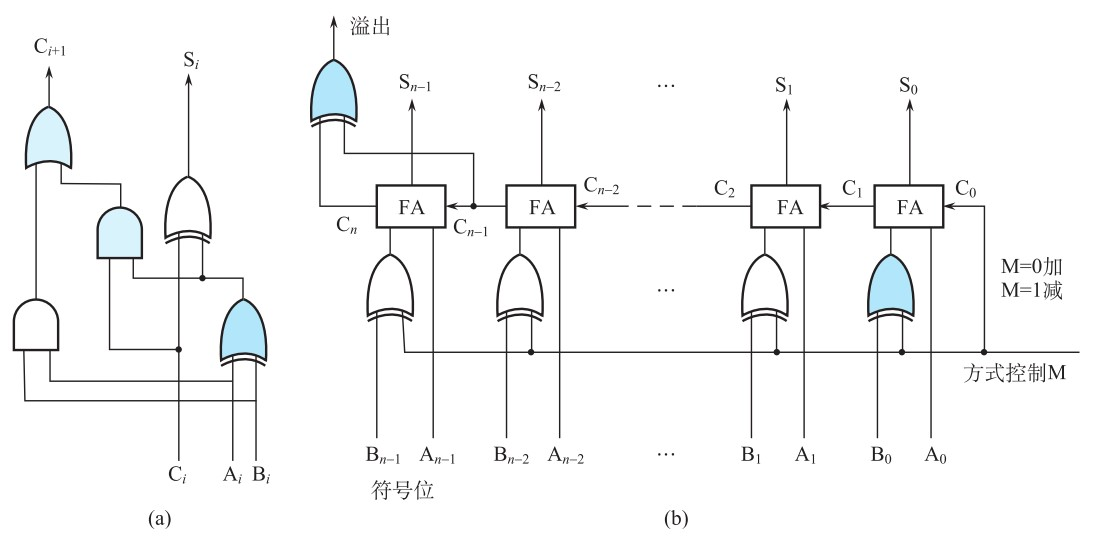​ 下面来分析延迟。在门电路分析中，我们选定信号通过与门或者或门的时间为$$T$$，且设经过异或门的时间为$$3T$$，则对于单个全加器而言，产生加和$$S_i$$的延迟为$$3T+3T=6T$$、产生进位$$C_{i+1}$$的延迟为$$3T+T+T=5T$$。对于较为复杂的门电路来说，要计算它的延迟就是要找到其中最长的时间链，对于上图的行波进位的加法减法器来说，最长的时间链就是从$$A_0$$$$B_0$$输入经过$$n$$次进位最终产生溢出标志的链路，该链路所花费的时间为

$3T(\text{对}B_0\text{预处理})+3T(A_0\text{和}B_0\text{的异或门})+n\cdot2T(n\text{次进位})+3T(\text{产生溢出符号的异或门})=(2n+9)T$

\begin{aligned} C_1&=G_0+P_0C_0\\ C_2&=G_1+P_1C_1=G_1+P_1G_0+P_1P_0C_0\\ C_3&=G_2+P_2C_2=G_2+P_2G_1+P_2P_1G_0+P_2P_1P_0C_0\\ C_4&=G_3+P_3C_3=G_3+P_3G_2+P_3P_2G_1+P_3P_2P_1G_0+P_3P_2P_1P_0C_0\\ \cdots \end{aligned}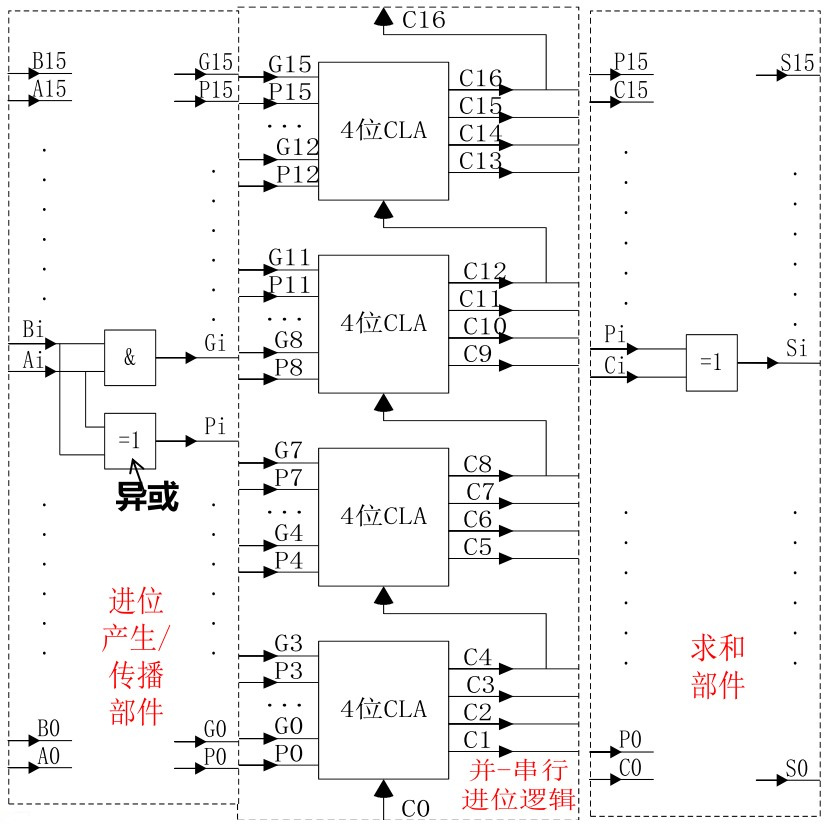$3T(\text{生成}P_0\text{的异或门})+2T(\text{产生}C_4\text{的时间})+2T(\text{产生}C_8\text{的时间})+2T(\text{产生}C_{12}\text{的时间})\\ +2T(\text{产生}C_{16}\text{的时间})+3T(\text{最后生成}S_{15}\text{的异或门的时间})=14T$

$3T(\text{对}B_0\text{预处理})+3T(A_0\text{和}B_0\text{的异或门})+(16-1)\cdot2T(15\text{次进位})=(2\cdot15n+9)T=39T$

### 2.4 定点乘法（原码）

​ 由于原码与真值极为相似（只差一个符号），而乘积的符号又可以通过两数符号的异或得到，因此，对于原码的乘法而言，只需要忽略符号位进行典型的二进制乘法，最后在结果中单独添加一个符号位即可，下面通过一个例子来说明：

### 2.5 定点乘法（原码）的门电路

​ 下面讨论不带符号（即无符号数，原码中不含符号位的部分的乘法也符合此情况）的阵列乘法器，不带符号的阵列乘法器的门电路为：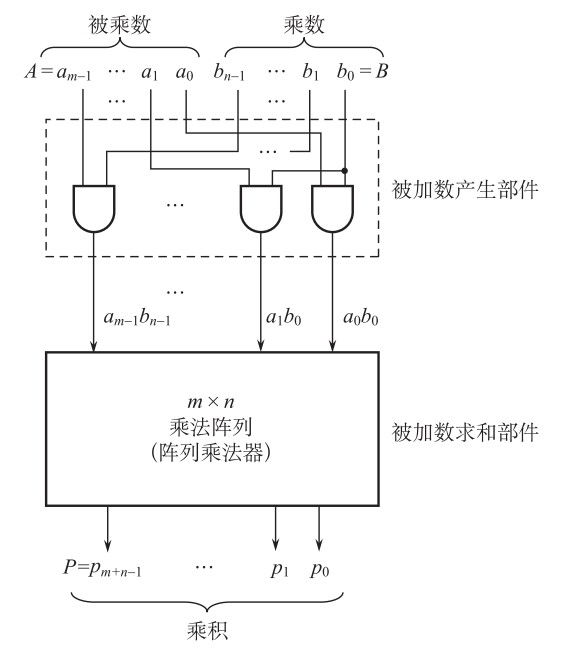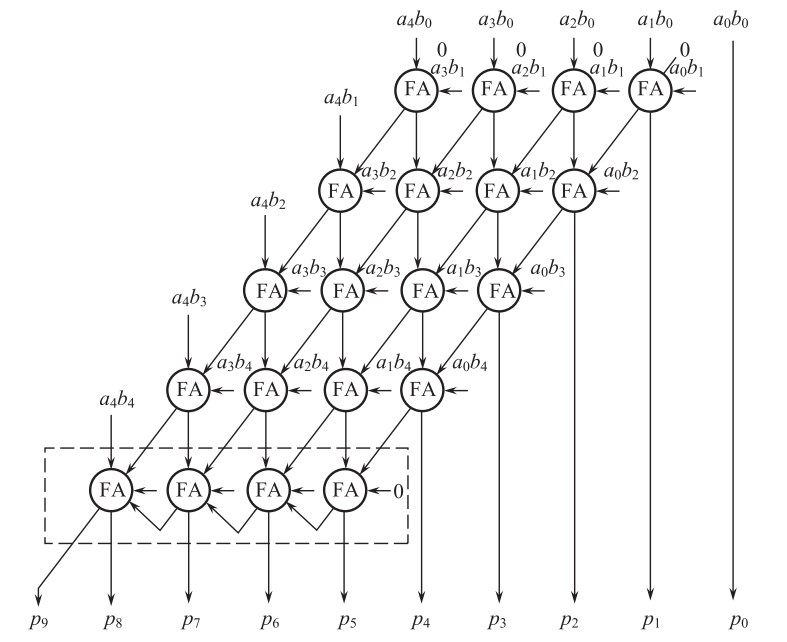$T(\text{生成被加数的时间})+(n-2)\cdot6T(\text{竖直向下的传递时间})+5T(\text{沿斜线向最后一行的进位时间})\\ +(n-2)\cdot2T(\text{最后一行内部经过}n-2\text{次进位到达最左侧全加器的时间})+3T(\text{生成}p_8\text{的一个异或门时间})\\ =(8n-7)T,\qquad33T(\text{上述}n=5\text{时})$

​ 如果我们输入的是两个原码，那么根据上面的不带符号的阵列乘法器，我们已经计算得到了不含符号位的乘法结果（比如输入$$-3$$$$5$$时，得到的是$$15$$），如果要使得到的结果也是补码表示，那么在所得结果之前加上两个乘数的符号位的异或即可。

### 2.6 定点乘法（补码）

​ 对于补码形式的数而言，它的乘法规则不再显然，通常我们先将这两个数转换为原码形式（通过后面所述的求补器实现），再按照原码的定点乘法规则（符号位单独考虑）进行乘法，在得到的乘法结果前面加上单独考虑的符号位，得到了原码表示的结果。如果想让最后的结果以补码形式表示，则只需要在最后使其通过求补器即可。下面通过两个例子说明该思路的具体操作过程：

​ 上面这种将补码转换为原码的方法，在思路上很简便，将补码的情况转变成了我们在2.4节中讨论过的情况。下面介绍直接使用补码进行乘法的思路，该思路不需要转换为原码，一定程度上减少了出错的可能性。

​ 补码不能直接参与乘法的原因是：符号位的含义与后面的一般数码没有统一性，参考资料中唐朔飞著作中提到了当两个乘数的补码形式的符号任意时的Booth算法，此处略去不谈。

### 2.7 定点乘法（补码）的门电路

​ 之前现在我们已经讨论了原码的乘法器实现，如果试图计算的是两个补码的乘法结果，那么对于输入的补码而言，根据2.6节的内容，先将这两个数转换为原码形式（通过后面所述的求补器实现），再按照原码的定点乘法规则（符号位单独考虑）进行乘法，在得到的乘法结果前面加上单独考虑的符号位，得到了原码表示的结果。如果想让最后的结果以补码形式表示，则只需要在最后使其通过求补器即可，门电路如下所示：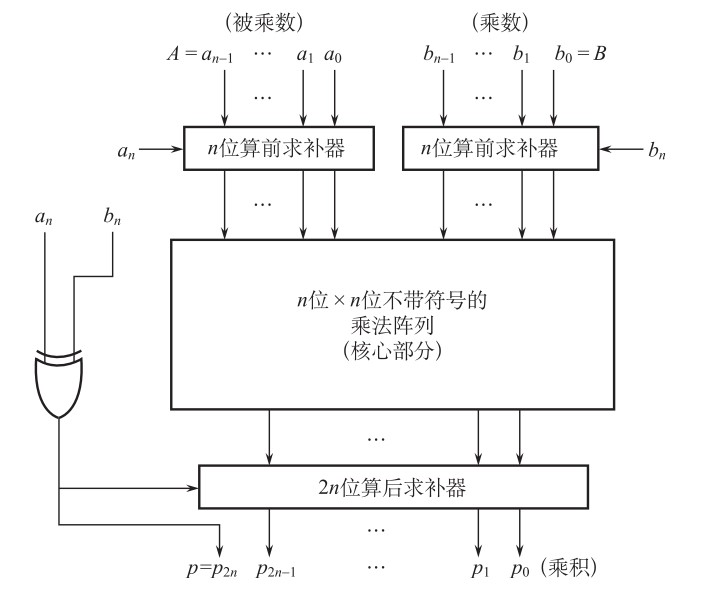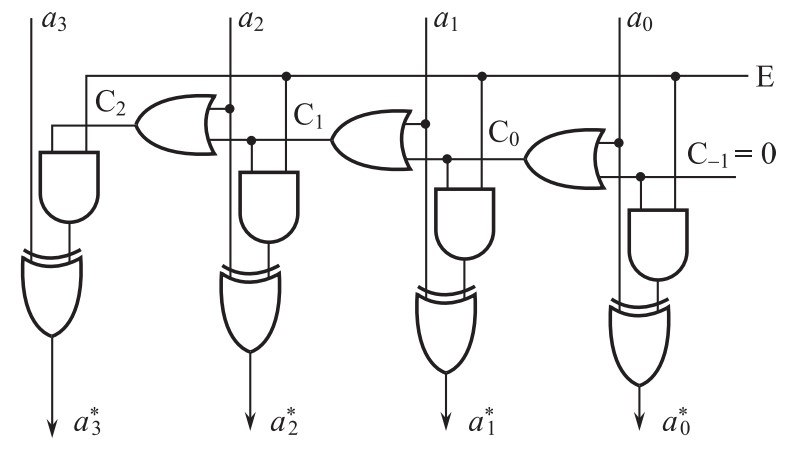$$E=1$$时进行求补运算，当$$E=0$$时输入和输出一样。该器件实现的思路为：从数的最右端$$a_0$$开始，由右向左，直到找出第一个$$1$$，例如$$a_i=1(0\leq i\leq n)$$。这样，$$a_i$$以右的每一个输入位，包括$$a_i$$自己，都保持不变，而$$a_i$$以左的每一个输入位都求反。鉴于此，横向链式线路中的第$$i$$扫描级的输出$$C_i$$$$1$$的条件是：第$$i$$级的输入位$$a_i=1$$，或者第$$i$$级链式输入（来自右起前$$i–1$$级的链式输出）$$C_i–1=1$$。另外，最右端的起始链式输入$$C_{–1}$$必须永远置成$$0$$。如果我们有$$n+1=5$$位的输入，由于符号位单独考虑，则该求补器中最长的链路为：从$$C_{-1}$$开始、经过$$(n+1)-1$$次进位、再经过一个与门和一个异或门输出$$a_3^*$$的链路，因此延迟为

$((n+1)-1)\cdot2T(n-1\text{次进位})+2T(与门)+3T(异或门)=(2n+5)T,\quad13T(\text{上述}n+1=5\text{时})$

​ 最后指出，由于补码的唯一性，我们既可以对补码求补得到原码（符号位单独考虑），也可以对补码求补得到原码（符号位单独考虑）。

### 2.8 定点除法（原码）

​ 先来讨论原码的除法。由于在二进制除法中商数只可能是$$1$$，故余数减去一倍的除数只有两种情况：够减（减法结果大于零，此时令商为$$1$$）与不够减（减法结果小于零，此时令商为$$0$$），在实际应用中，有两种具体的思路：

• 恢复余数法：当不够减的时候，恢复余数，并上商为$$0$$，进行后续的操作，这种思路最后得到的余数是准确的
• 加减交替法：够减则下一步进行减法、不够减则下一步进行加法，这种思路最后得到的余数可能需要纠正

\begin{aligned} \text{若}r_i'=2r_{i-1}-y>0&\quad\text{则}r_i=r_i'\text{且}r_{i+1}'=2r_i-y=2r_i'-y\\ \text{若}r_i'=2r_{i-1}-y<0&\quad\text{则}r_i=r_i'+y\text{且}r_{i+1}'=2r_i-y=2r_i'+y \end{aligned}

​ 上面讨论的原码除法，适用于任何原码之间的除法（正数与正数、正数与负数、负数与正数、负数与负数，中间两种的符号位单独处理），虽然称为原码乘法，但运算中涉及到的加减法仍然是补码的，所谓“原码”仅是指参与除法的两个数需要先取绝对值才能才与运算，至于商数的符号位则单独确定，这和原码乘法是类似的，但不同之处在于，原码乘法中不涉及到减法而原码除法涉及到减法。

​ 我们在此只讨论了原码（即无符号数，原码中不含符号位的部分的乘法也符合此情况）的除法原理，有符号数（比如补码）的情况后面再谈。后面的例子说明这一点。下面通过两个例子说明原码定点除法的实现过程：

$$i$$ 被除数 除数 $$2r_{i-1}'$$ $$r_i'=2r_{i-1}'\pm y$$
$$1$$ $$0~1001$$ $$0~1011$$ 被除数 $$\text{被除数}-0~1011=0~1001+1~0101=1~1110<0$$ $$0$$
$$2$$ $$0~1001$$ $$0~1011$$ $$1~1100$$ $$1~1100+0~1011=1~0~0111$$，丢弃第一位得$$0~0111>0$$ $$1$$
$$3$$ $$0~1001$$ $$0~1011$$ $$0~1110$$ $$0~1110-0~1011=1~0~0011$$，丢弃第一位得$$0~0011>0$$ $$1$$
$$4$$ $$0~1001$$ $$0~1011$$ $$0~0110$$ $$0~0110-0~1011=1~1011<0$$ $$0$$
$$5$$ $$0~1001$$ $$0~1011$$ $$1~0110$$ $$1~0110+0~1011=1~0~0001$$，丢弃第一位得$$0~0001>0$$ $$1$$
$$\cdots$$ $$0~1001$$ $$0~1011$$ $$\cdots$$ $$\cdots$$ $$\cdots$$

$$i$$ 被除数$$a$$ 除数$$b$$ $$2r_{i-1}'$$ $$r_i'=2r_{i-1}'\pm y$$
$$1$$ $$0~1001$$ $$0~1011$$ 被除数 $$\text{被除数}-0~1011=0~1001+1~0101=1~1110<0$$ $$0$$
$$2$$ $$0~1001$$ $$0~1011$$ $$1~1100$$ $$1~1100+0~1011=1~0~0111$$，丢弃第一位得$$0~0111>0$$ $$1$$
$$3$$ $$0~1001$$ $$0~1011$$ $$0~1110$$ $$0~1110-0~1011=1~0~0011$$，丢弃第一位得$$0~0011>0$$ $$1$$
$$4$$ $$0~1001$$ $$0~1011$$ $$0~0110$$ $$0~0110-0~1011=1~1011<0$$ $$0$$
$$5$$ $$0~1001$$ $$0~1011$$ $$1~0110$$ $$1~0110+0~1011=1~0~0001$$，丢弃第一位得$$0~0001>0$$ $$1$$
$$\cdots$$ $$0~1001$$ $$0~1011$$ $$\cdots$$ $$\cdots$$ $$\cdots$$

​ 下面来看纠正余数的问题。采用加减交替法的原码除法的一个小缺陷就是最后得到的余数可能不是真正的余数（但最后得到的商数一定是真的商数）。原码除法的余数纠正并不是明晰的，因为它涉及到原码和补码的转换，我们将在后面补码除法除法中详细说明余数纠正的一般规则。

### 2.9 定点除法（原码）的门电路

​ 从上面的内容，我们不难发现，原码的定点除法具有下面的特征：

• 在形式上永远是正数和正数的除法（因为取了绝对值）
• 被除数不需要在计算过程中保存，除数需要在计算过程中保存并向下一级传递
• 每次计算的可能余数$$r_i'$$在参与下一级运算之前需要左移一位（该操作模拟的是真实除法过程中在余数后面加上一个$$0$$的过程）
• 由于通过加减交替的思路实现，故内部应该整合一个进行补码加减法的门电路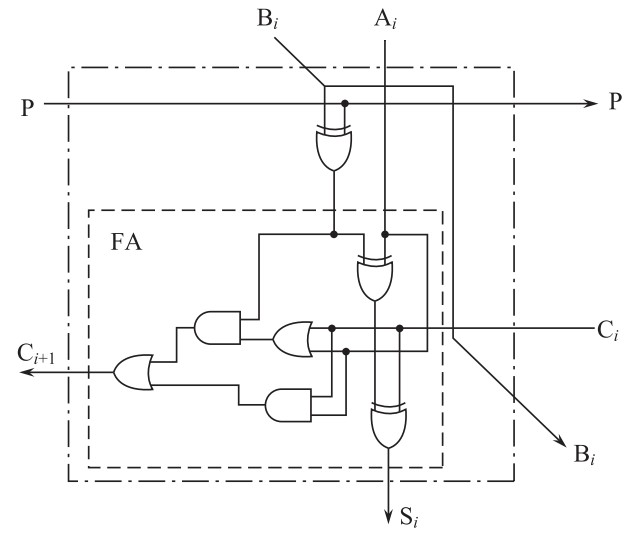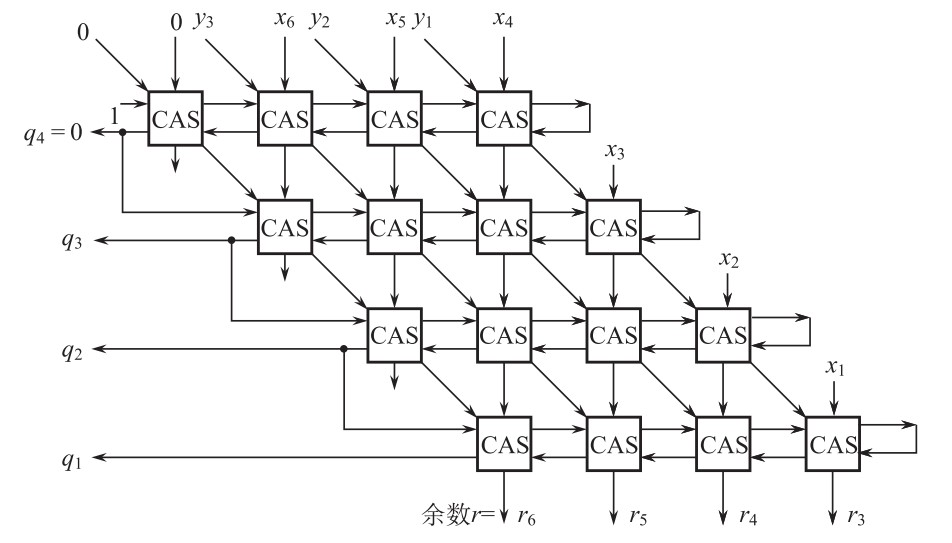（最后得到的是不含符号位的商）。其中，沿着斜线输入的$$0y_3y_2y_1$$为除数，沿着竖线输入的$$0x_6x_5x_4x_3x_2x_1$$为被除数。对于第一行而言，第一次执行的操作为减法，故输入$$P=1$$（且第一行最右侧输入的$$C_{-1}=P=1$$），第一行最左侧的进位输出用于判断可能余数的正负（由此决定下一次进行加法还是减法），如果进位为$$0$$则说明可能余数为负数（此时令商为$$0$$且下一次进行加法），如果进位为$$1$$则说明可能余数为正数（此时令商为$$1$$且下一次进行减法）。从例$$11$$也可以看出，正数总是通过丢弃最高位才得到的，这实际上由更深入的原理来保证。在严格的$$4$$位除$$4$$位的除法当中，被除数$$0x_6x_5x_4x_3x_2x_1=0x_6x_5x_4000$$，在除法向下推进的过程中，上一次得到的可能余数的最高位不再参与运算，并在最低位引入$$x_3$$$$x_2$$$$x_1$$，这满足了可能余数$$r_i'$$在参与下一级运算之前需要左移一位的要求。

​ 现在来分析延迟。在上述所示的$$4$$位除$$4$$位的阵列除法器中，我们记真正有效的位数$$3$$$$n$$，则被除数有效部分为$$2n$$，除数有效部分为$$n$$，阵列除法器一共有$$(n+1)^2$$个 CAS 单元。该阵列除法器中最长的链路为：从$$P=1$$输入开始，经过每一行的每一次进位再进入下一行、直到最后生成最后一个商$$p_1$$的时间，因此延迟为

$(n+1)^2\cdot3T((n+1)^2\text{次进位时间})=3(n+1)^2T,\quad48T(\text{上述}n=3\text{时})$

### 2.10 定点除法（补码）

​ 现在略去具体的推导（详见参考资料中唐朔飞著作），直接给出补码除法的一般规则：

$$[x]_{\text{补}}$$$$[y]_{\text{补}}$$ $$[R]_{\text{补}}$$$$[y]_{\text{补}}$$ 上商 下一步

$$i$$ $$[x]_{\text{补}}$$ $$[y]_{\text{补}}$$ $$2r_{i-1}'$$ $$r_i'=2r_{i-1}'\pm y$$
$$1$$ $$1~0111$$ $$0~1101$$ 被除数 $$1~0111+0~1101=1~0~0100$$，丢弃第一位得$$0~0100$$$$[y]_{\text{补}}$$同号 $$1$$
$$2$$ $$1~0111$$ $$0~1101$$ $$0~1000$$ $$0~1000-0~1101=1~1011$$，与$$[y]_{\text{补}}$$异号 $$0$$
$$3$$ $$1~0111$$ $$0~1101$$ $$1~0110$$ $$1~0110+0~1101=1~0~0011$$，丢弃第一位得$$0~0011$$$$[y]_{\text{补}}$$同号 $$1$$
$$4$$ $$1~0111$$ $$0~1101$$ $$0~0110$$ $$0~0110-0~1101=1~1001$$，与$$[y]_{\text{补}}$$异号 $$0$$
$$\cdots$$ $$1~0111$$ $$0~1101$$ $$\cdots$$ $$\cdots$$ $$\cdots$$

$$i$$ $$[x]_{\text{补}}$$ $$[y]_{\text{补}}$$ $$2r_{i-1}'$$ $$r_i'=2r_{i-1}'\pm y$$
$$1$$ $$0~1001$$ $$0~1101$$ 被除数 $$0~1001-0~1101=1~1100$$，与$$[y]_{\text{补}}$$异号 $$0$$
$$2$$ $$0~1001$$ $$0~1101$$ $$1~1000$$ $$1~1000+0~1101=1~0~0101$$，丢弃第一位得$$0~0101$$$$[y]_{\text{补}}$$同号 $$1$$
$$3$$ $$0~1001$$ $$0~1101$$ $$0~1010$$ $$0~1010-0~1101=1~1101$$，与$$[y]_{\text{补}}$$异号 $$0$$
$$4$$ $$0~1001$$ $$0~1101$$ $$1~1010$$ $$1~1010+0~1101=1~0~0111$$，丢弃第一位得$$0~0111$$$$[y]_{\text{补}}$$同号 $$1$$
$$\cdots$$ $$0~1001$$ $$0~1101$$ $$\cdots$$ $$\cdots$$ $$\cdots$$

​ 下面来看余数的纠正：

• 如果当前上商为$$1$$，则当前余数就是真余数
• 如果当前上商为$$0$$，则当前余数需要加上$$[y]_{\text{补}}$$才是真余数

• 上面第一个例，$$i=4$$时，上商$$0$$，真余数为$$0~0110$$
• 上面第一个例，$$i=3$$时，上商$$1$$，真余数为$$0~0011$$
• 上面第二个例，$$i=4$$时，上商$$1$$，真余数为$$1~1010$$
• 上面第二个例，$$i=3$$时，上商$$0$$，真余数为$$0~1010$$

### 2.11 定点除法（补码）的门电路

​ 结构和原码的除法门电路一样，只不过涵盖了符号位，且检测上商的时候不仅需要可能余数$$[R]_{\text{补}}$$的符号位、而且需要除数$$[y]_{\text{补}}$$，这两者同号则上商$$1$$，否则上商$$0$$。另外，原码除法必定是正数除以正数，所以第一步一定进行的是减法，但在补码除法中如果被除数和除数同号则第一步进行减法、否则进行加法，所以首先输入的$$P$$可能是$$1$$（进行减法）、也可能是$$0$$（进行加法）。

## 5 浮点运算

### 5.1 浮点加减法

​ 完成浮点加减法的大致过程分为四步：

• $$0$$操作数检查
• 比较阶码大小并完成对阶
• 尾数进行加减运算
• 结果规格化并进行舍入处理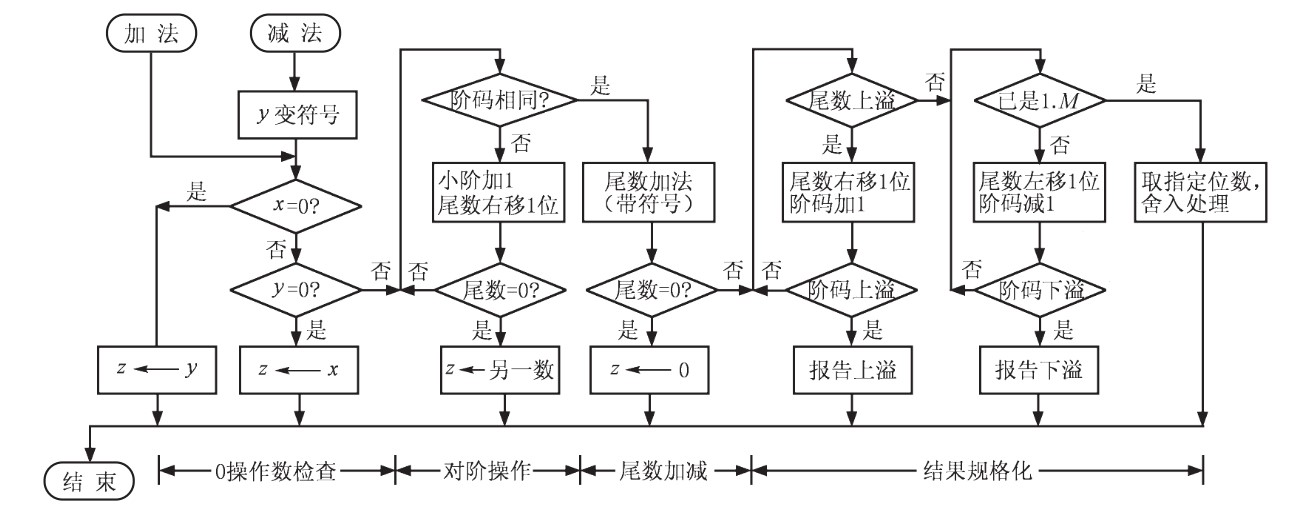• 就近舍入：就是”四舍五入“。例如，若被舍弃的五位二进制位为$$10010$$，则丢弃这五位之后的最低二进制位因该加一；若被舍弃的四位二进制位为$$0111$$，则丢弃这四位之后的最低二进制位保持不变
• $$0$$舍入：即朝数轴原点方向舍入，就是简单的截尾。无论尾数是正数还是负数，截尾都使取值的绝对值比原值的绝对值小。这种方法容易导致误差累积
• $$+\infty$$舍入：对正数来说，只要多余位不全为$$0$$则向最低有效位进$$1$$；对负数来说，则是简单的截尾
• $$-\infty$$舍入：对负数来说，只要多余位不全为$$0$$则向最低有效位进$$1$$；对正数来说，则是简单的截尾

• 阶码上溢：超过了阶码可能表示的最大值的正整数值，一般将其认为是$$+\infty$$$$-\infty$$
• 阶码下溢：超过了阶码可能表示的最小值的负指数值，一般将其认为是$$0$$

• 尾数上溢：两个同符号尾数相加产生了最高位向上的进位，要将尾数右移，阶码增$$1$$来重新对齐
• 尾数下溢：在将尾数右移时，尾数的最低有效位从尾数域右端流出，要进行舍入处理

### 5.2 浮点乘除法

​ 浮点乘数法的计算过程比浮点加减法的计算过程从概念上来说简单许多，大体上分为六个步骤：

• $$0$$操作数检查
• 阶码加减操作
• 尾数乘除操作
• 结果规格化
• 舍入处理
• 确定积的符号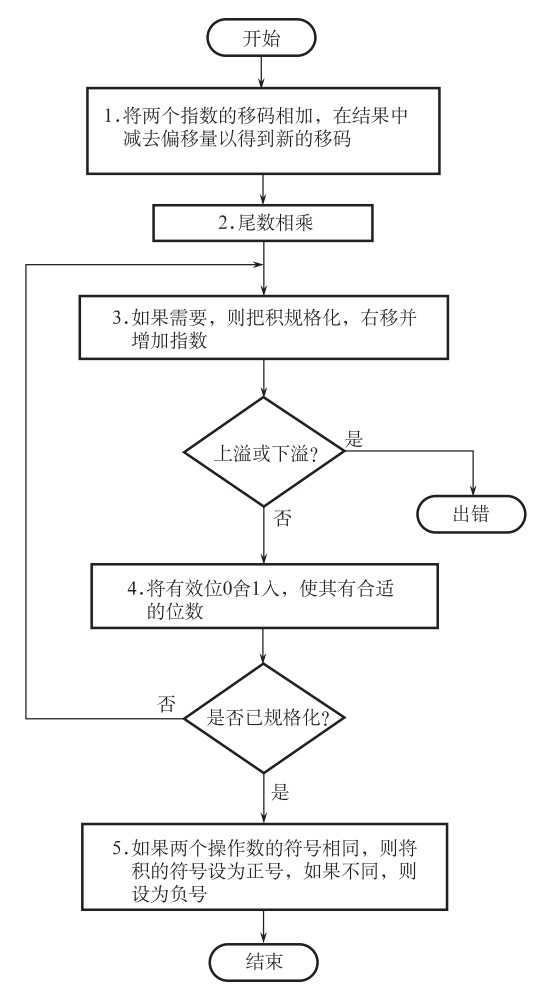## 参考资料

• 白中英，戴志涛，《计算机组成原理（第六版）》，科学出版社，2019
• 白中英，杨春武，《计算机组成原理：题解、题库与试验（第三版）》，科学出版社，2001
• 唐朔飞，《计算机组成原理（第二版）》，高等教育出版社，2008
• Randal E. Bryant, David R. O’Hallaron, Computer Systems - A Programmer’s Perspective 3 Edi Global Edition, Pearson, 2016
• David A. Patterson, John L. Hennessy, Computer Organization and Design: The Hardware/Software Interface 5 Edi, Morgan Kaufmann, 2013posted @ 2022-04-04 18:10  faranten  阅读(518)  评论(0编辑  收藏  举报